# ISEE Lower Level Quantitative : How to subtract

## Example Questions

### Example Question #387 : Isee Lower Level (Grades 5 6) Quantitative Reasoning

The dog’s body is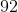centimeters long and his tail is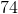centimeters long. How much longer is his body than his tail?centimeterscentimeterscentimeterscentimeterscentimeterscentimeters

Explanation:

This is a subtraction problem because we want to know how much longer the dog's body is compared to his tail, or the difference in their length.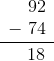### Example Question #388 : Isee Lower Level (Grades 5 6) Quantitative Reasoning

The coffee cup is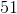centimeters tall and the water glass iscentimeters tall. How much taller is the water glass than the coffee cup?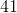centimeterscentimeterscentimeterscentimeterscentimeterscentimeters

Explanation:

This is a subtraction problem because we want to know how much taller the water glass is compared to the coffee cup, or the difference in their height.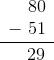### Example Question #41 : How To Subtract

The cat isinches long and the dog isinches long. How much longer is the dog than the cat?inchesinchesinchesinchesinchesinches

Explanation:

This is a subtraction problem because we want to know how much longer the dog is compared to the cat, or the difference between their lengths.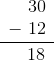### Example Question #390 : Isee Lower Level (Grades 5 6) Quantitative Reasoning

On the morning of December 24th, a tree lot hadtrees left to sell. By the end of the day, the lot only hadtrees left. How many trees were sold?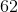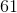Explanation:

This is a subtraction problem because we want to know how many trees were taken away, or sold, from the total. We take the number of trees they started with, and then subtract the number of trees they have left to find out how many they sold.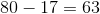.

### Example Question #56 : How To Subtract

Spot has a box of treats withbones left. The box started off withtreats. How many treats has Spot eaten from the box?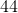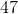Explanation:

This is a subtraction problem because we want to know how many of the total treats Spot has eaten. We take the total number of treats and then subtract the number of treats that are left in the box.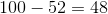.

### Example Question #57 : How To Subtract

The baseball team has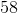balls to practice with. There areboys on the team. If they each take a ball, how many balls are left over?Explanation:

This is a subtraction problem because we want to know how many balls are left over after each boy takes one. We start with the number of balls and then subtract the number that was taken.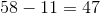.

### Example Question #58 : How To Subtract

Jenny hasgames. She let her friend useof them for her birthday part. How many does Jenny have left?Explanation:

This is a subtraction problem because we want to know how many games Jenny has left after she gives some to her friend. We take the total number of games that Jenny has, and then we subtract the number that she gave to her friend.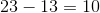.

### Example Question #59 : How To Subtract

Sarah gotbirthday gifts for her birthday. She’s openedof them. How many does she have left to open?Explanation:

This is a subtraction problem because we want to know how many gifts she has left to open after she’s already opened some of her gifts. We take the total number of gifts she has, and then we subtract the number of gifts that she’s opened.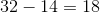.

### Example Question #60 : How To Subtract

Matt invitedof his friends to a cookout.of his friends did not come. How many of his friends did come to his cookout?Explanation:

This is a subtraction problem because we want to find out how many of Matt’s invited friends were able to come to his party. We take the total number of people that were invited, and subtract the number of people that did not come.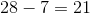### Example Question #391 : Isee Lower Level (Grades 5 6) Quantitative Reasoning

Ms. Smith’s class hasstudents.of her students are out with the flu today. How many students are in the class today?This is a subtraction problem because we want to know how many students in Ms. Smith’s class are in school today out of the total number of students that she has. We take the total number of students and subtract the number of students who are not in school today.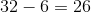.